Question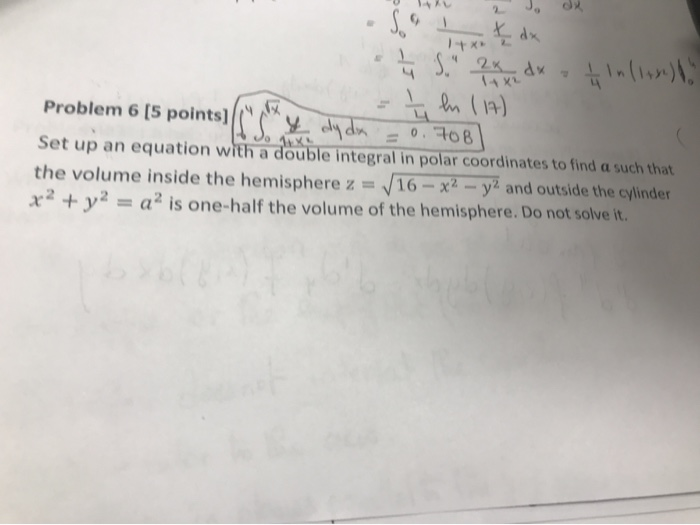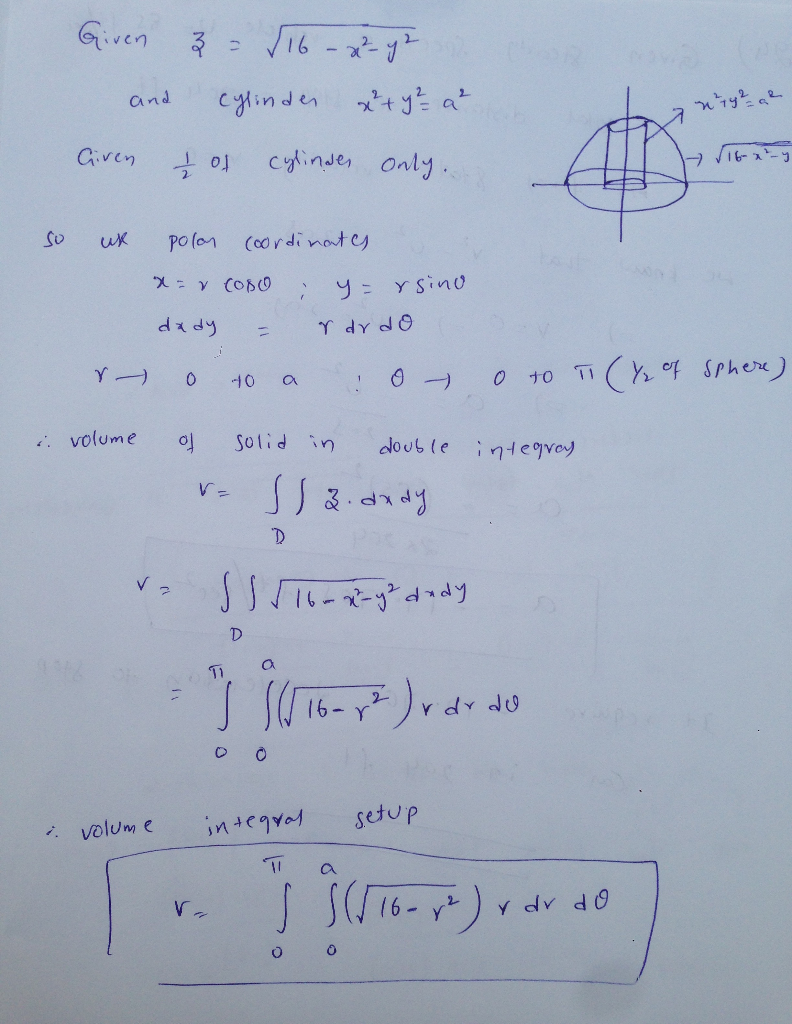#### Earn Coins

Coins can be redeemed for fabulous gifts.

Similar Homework Help Questions
• ### 4. (14 points) Using polar coordinates, set up, but DO NOT EVALUATE, a double integral to find the volume of the solid region inside the cylinder x2 +(y-1)2-1 bounded above by the surface z=e-/-/...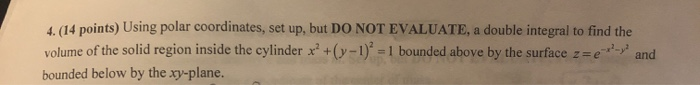4. (14 points) Using polar coordinates, set up, but DO NOT EVALUATE, a double integral to find the volume of the solid region inside the cylinder x2 +(y-1)2-1 bounded above by the surface z=e-/-/ and bounded below by the xy-plane. 4. (14 points) Using polar coordinates, set up, but DO NOT EVALUATE, a double integral to find the volume of the solid region inside the cylinder x2 +(y-1)2-1 bounded above by the surface z=e-/-/ and bounded below by the xy-plane.

• ### 6. (4 pts) Consider the double integral∫R(x2+y)dA=∫10∫y−y(x2+y)dxdy+∫√21∫√2−y2−√2−y2(x2+y)dxdy.(a) Sketch the region of integration R in Figure 3.(b)...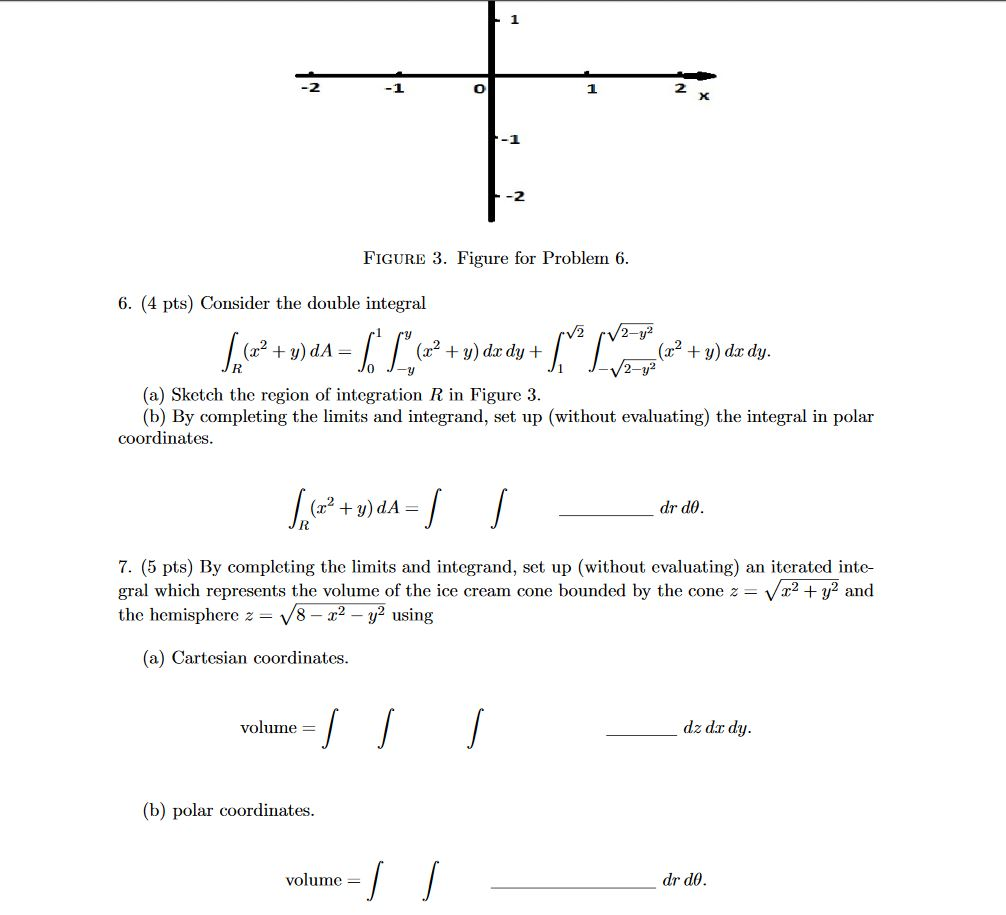6. (4 pts) Consider the double integral∫R(x2+y)dA=∫10∫y−y(x2+y)dxdy+∫√21∫√2−y2−√2−y2(x2+y)dxdy.(a) Sketch the region of integration R in Figure 3.(b) By completing the limits and integrand, set up (without evaluating) the integral in polar coordinates.∫R(x2+y)dA=∫∫drdθ.7. (5 pts) By completing the limits and integrand, set up (without evaluating) an iterated inte-gral which represents the volume of the ice cream cone bounded by the cone z=√x2+y2andthe hemisphere z=√8−x2−y2using(a) Cartesian coordinates.volume =∫∫∫dz dxdy.(b) polar coordinates.volume =∫∫drdθ. -1 -2 FIGURE 3. Figure for Problem 6. 6. (4 pts)...

• ### 6. (4 pts) Consider the double integral∫R(x2+y)dA=∫10∫y−y(x2+y)dxdy+∫√21∫√2−y2−√2−y2(x2+y)dxdy.(a) Sketch the region of integrationRin Figure 3.(b) By completing...6. (4 pts) Consider the double integral∫R(x2+y)dA=∫10∫y−y(x2+y)dxdy+∫√21∫√2−y2−√2−y2(x2+y)dxdy.(a) Sketch the region of integrationRin Figure 3.(b) By completing the limits and integrand, set up (without evaluating) the integral in polar coordinates. -1 -2 FIGURE 3. Figure for Problem 6. 6. (4 pts) Consider the double integral V2 /2-y² + = (x2 + y) dx dy + + y) do dy. 2-y2 (a) Sketch the region of integration R in Figure 3. (b) By completing the limits and integrand, set up (without evaluating)...

• ### Consider the solid inside the hemispherez- 4-x2-y2, outside the cylinder x2+y2 -1 and y* , outside...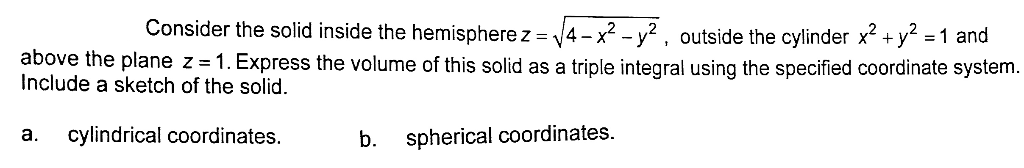Consider the solid inside the hemispherez- 4-x2-y2, outside the cylinder x2+y2 -1 and y* , outside the cylinder x' +y 1 an above the plane z 1. Express the volume of this solid as a triple integral using the specified coordinate systerm Include a sketch of the solid. a. cylindrical coordinates. b. spherical coordinates.

• ### (16) (7 points) Set up an iterated integral of f (x, y, z) = x2 +...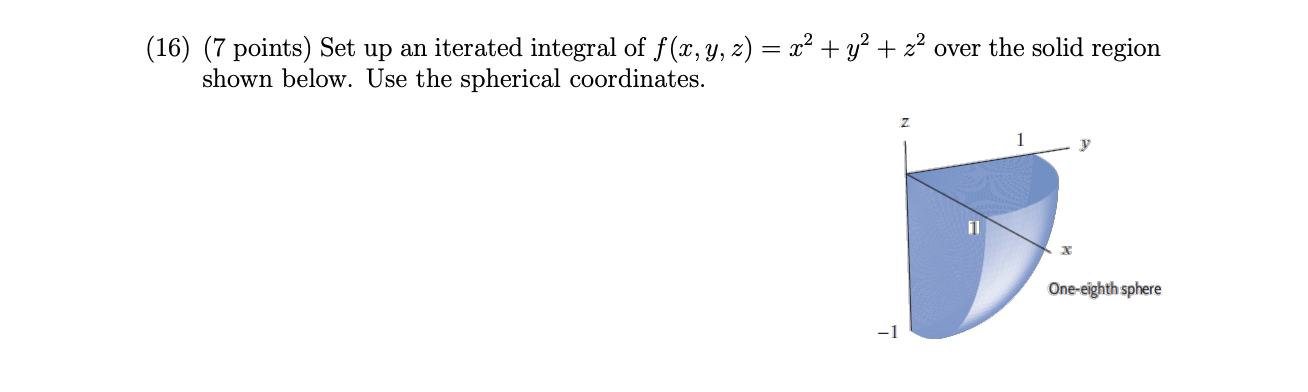(16) (7 points) Set up an iterated integral of f (x, y, z) = x2 + y2 + z2 over the solid region shown below. Use the spherical coordinates. N 1 y - One-eighth sphere

• ### Set up only b. Find the volume of the solid bounded by z x2 y2 and z 3 in spherical coordinates. Set-up only (OJ 7a. Change to spherical coordinates. Set-up only.X 2. f(x, y,z)dzdxdy b. Find fffe&...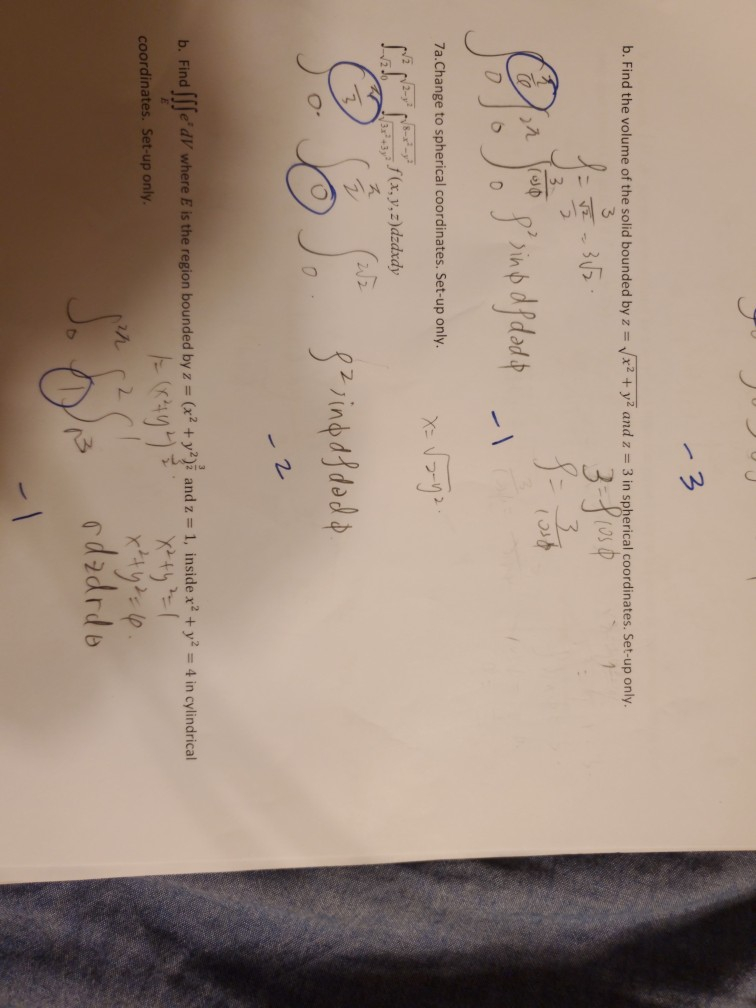Set up only b. Find the volume of the solid bounded by z x2 y2 and z 3 in spherical coordinates. Set-up only (OJ 7a. Change to spherical coordinates. Set-up only.X 2. f(x, y,z)dzdxdy b. Find fffe'd/where E is the region bounded by z (x2 + y2)2 and z 1, inside x2 + y2 4 in cylindrical coordinates. Set-up only b. Find the volume of the solid bounded by z x2 y2 and z 3 in spherical coordinates. Set-up only...

• ### Set up, but do not evaluate, a triple integral in cylindrical coordinates that gives the volume...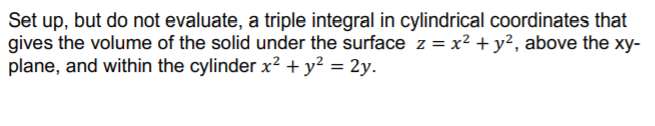Set up, but do not evaluate, a triple integral in cylindrical coordinates that gives the volume of the solid under the surface z = x2 + y2, above the xy- plane, and within the cylinder x2 + y2 = 2y.

• ### Question Use cylindrical coordinates to set up the triple integral needed to find the volume of...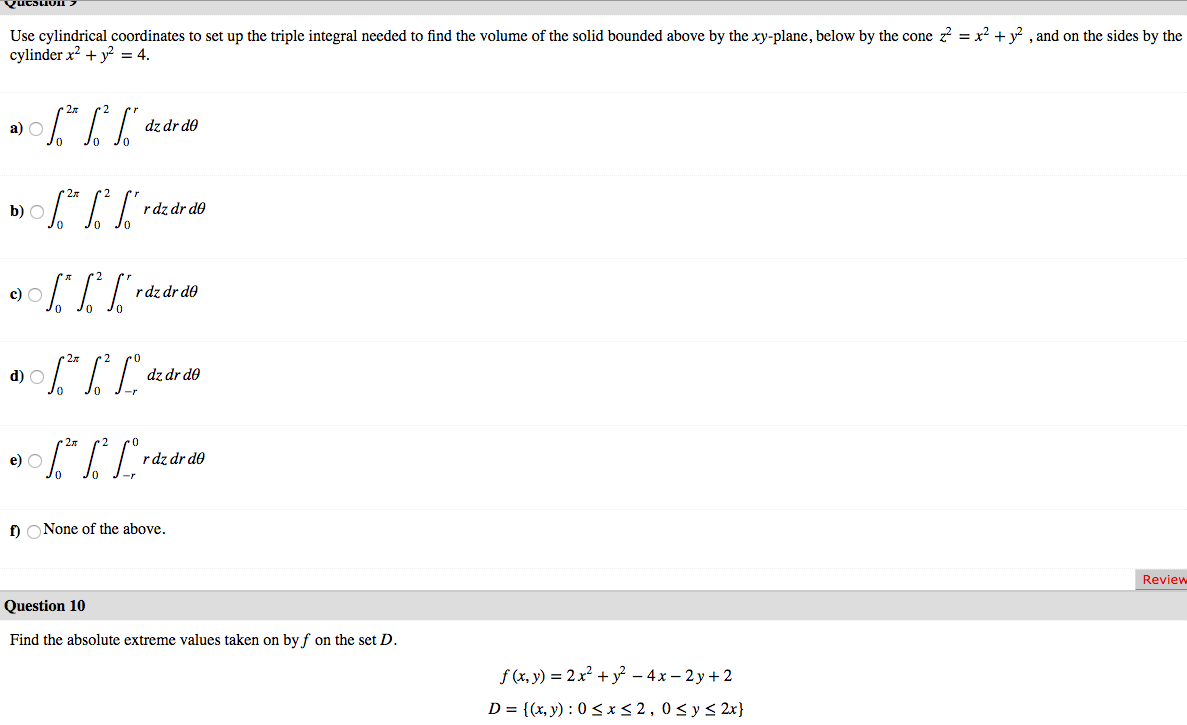Question Use cylindrical coordinates to set up the triple integral needed to find the volume of the solid bounded above by the xy-plane, below by the cone z = x2 + y2 , and on the sides by the cylinder x2 + y2 = 4. a) 06.* %* ["dz dr do b) \$* * S*rde de do JO 0% ] raz dr do a) \$** [Lºdz dr do 0906.*|*Lºrdz dr do 2 po dz dr do Jo J- O J-...

• ### 6. (4 pts) Consider the double integral∫R(x2+y)dA=∫10∫y−y(x2+y)dxdy+∫√21∫√2−y2−√2−y2(x2+y)dxdy.(a) Sketch the region of integration R in Figure 3.(b)...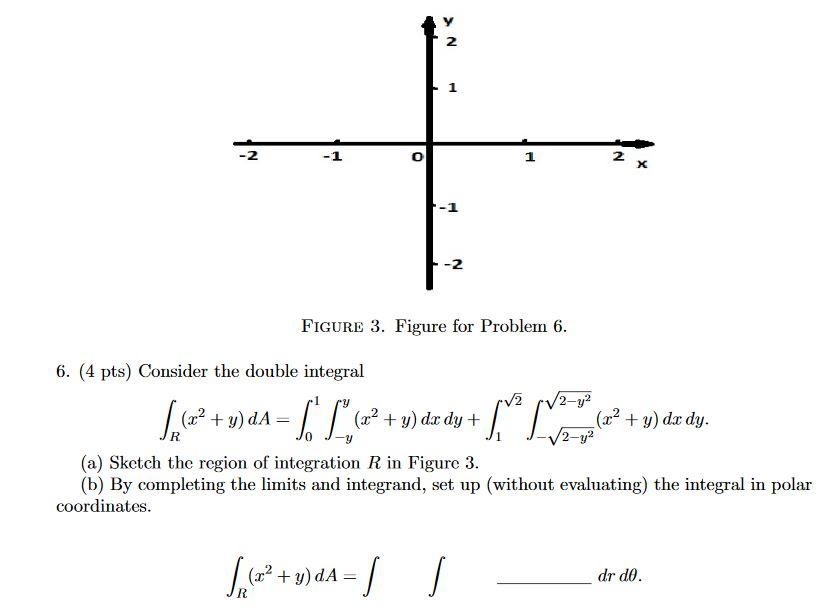6. (4 pts) Consider the double integral∫R(x2+y)dA=∫10∫y−y(x2+y)dxdy+∫√21∫√2−y2−√2−y2(x2+y)dxdy.(a) Sketch the region of integration R in Figure 3.(b) By completing the limits and integrand, set up (without evaluating) the integral in polar coordinates. 2 1 2 X -2 FIGURE 3. Figure for Problem 6. 6. (4 pts) Consider the double integral V2 2-y2 (2? + y) dA= (32 + y) dx dy + (x2 + y) dx dy. 2-y? (a) ketch the region of integration R in Figure 3. (b) By completing...

• ### 10. Consider the integral (x + y + z) dV where D is the volume inside...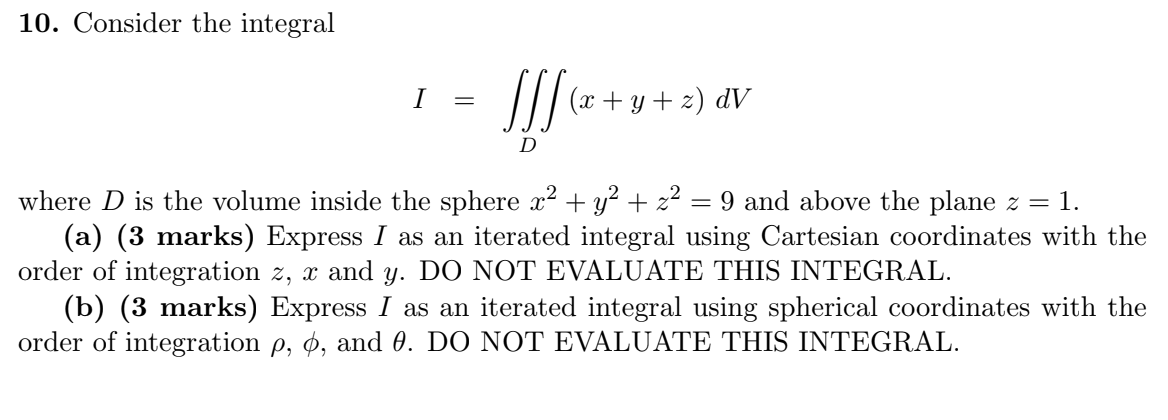10. Consider the integral (x + y + z) dV where D is the volume inside the sphere x2 + y2 + x2 = 9 and above the plane z = 1. (a) (3 marks) Express I as an iterated integral using Cartesian coordinates with the order of integration z, x and y. DO NOT EVALUATE THIS INTEGRAL. (b) (3 marks) Express I as an iterated integral using spherical coordinates with the order of integration p, 0, and 0. DO...